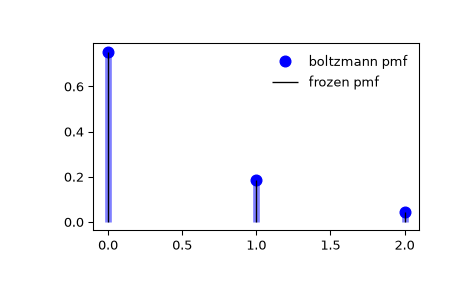# scipy.stats.boltzmann¶

scipy.stats.boltzmann = <scipy.stats._discrete_distns.boltzmann_gen object>[source]

A Boltzmann (Truncated Discrete Exponential) random variable.

As an instance of the rv_discrete class, boltzmann object inherits from it a collection of generic methods (see below for the full list), and completes them with details specific for this particular distribution.

Notes

The probability mass function for boltzmann is:

$f(k) = (1-\exp(-\lambda)) \exp(-\lambda k) / (1-\exp(-\lambda N))$

for $$k = 0,..., N-1$$.

boltzmann takes $$\lambda > 0$$ and $$N > 0$$ as shape parameters.

The probability mass function above is defined in the “standardized” form. To shift distribution use the loc parameter. Specifically, boltzmann.pmf(k, lambda_, N, loc) is identically equivalent to boltzmann.pmf(k - loc, lambda_, N).

Examples

>>> from scipy.stats import boltzmann
>>> import matplotlib.pyplot as plt
>>> fig, ax = plt.subplots(1, 1)


Calculate the first four moments:

>>> lambda_, N = 1.4, 19
>>> mean, var, skew, kurt = boltzmann.stats(lambda_, N, moments='mvsk')


Display the probability mass function (pmf):

>>> x = np.arange(boltzmann.ppf(0.01, lambda_, N),
...               boltzmann.ppf(0.99, lambda_, N))
>>> ax.plot(x, boltzmann.pmf(x, lambda_, N), 'bo', ms=8, label='boltzmann pmf')
>>> ax.vlines(x, 0, boltzmann.pmf(x, lambda_, N), colors='b', lw=5, alpha=0.5)


Alternatively, the distribution object can be called (as a function) to fix the shape and location. This returns a “frozen” RV object holding the given parameters fixed.

Freeze the distribution and display the frozen pmf:

>>> rv = boltzmann(lambda_, N)
>>> ax.vlines(x, 0, rv.pmf(x), colors='k', linestyles='-', lw=1,
...         label='frozen pmf')
>>> ax.legend(loc='best', frameon=False)
>>> plt.show()Check accuracy of cdf and ppf:

>>> prob = boltzmann.cdf(x, lambda_, N)
>>> np.allclose(x, boltzmann.ppf(prob, lambda_, N))
True


Generate random numbers:

>>> r = boltzmann.rvs(lambda_, N, size=1000)


Methods

 rvs(lambda_, N, loc=0, size=1, random_state=None) Random variates. pmf(k, lambda_, N, loc=0) Probability mass function. logpmf(k, lambda_, N, loc=0) Log of the probability mass function. cdf(k, lambda_, N, loc=0) Cumulative distribution function. logcdf(k, lambda_, N, loc=0) Log of the cumulative distribution function. sf(k, lambda_, N, loc=0) Survival function (also defined as 1 - cdf, but sf is sometimes more accurate). logsf(k, lambda_, N, loc=0) Log of the survival function. ppf(q, lambda_, N, loc=0) Percent point function (inverse of cdf — percentiles). isf(q, lambda_, N, loc=0) Inverse survival function (inverse of sf). stats(lambda_, N, loc=0, moments=’mv’) Mean(‘m’), variance(‘v’), skew(‘s’), and/or kurtosis(‘k’). entropy(lambda_, N, loc=0) (Differential) entropy of the RV. expect(func, args=(lambda_, N), loc=0, lb=None, ub=None, conditional=False) Expected value of a function (of one argument) with respect to the distribution. median(lambda_, N, loc=0) Median of the distribution. mean(lambda_, N, loc=0) Mean of the distribution. var(lambda_, N, loc=0) Variance of the distribution. std(lambda_, N, loc=0) Standard deviation of the distribution. interval(alpha, lambda_, N, loc=0) Endpoints of the range that contains fraction alpha [0, 1] of the distribution